GMAT  >  Test: Ratio And Proportion- 1

# Test: Ratio And Proportion- 1

Test Description

## 15 Questions MCQ Test Quantitative for GMAT | Test: Ratio And Proportion- 1

Test: Ratio And Proportion- 1 for GMAT 2023 is part of Quantitative for GMAT preparation. The Test: Ratio And Proportion- 1 questions and answers have been prepared according to the GMAT exam syllabus.The Test: Ratio And Proportion- 1 MCQs are made for GMAT 2023 Exam. Find important definitions, questions, notes, meanings, examples, exercises, MCQs and online tests for Test: Ratio And Proportion- 1 below.
Solutions of Test: Ratio And Proportion- 1 questions in English are available as part of our Quantitative for GMAT for GMAT & Test: Ratio And Proportion- 1 solutions in Hindi for Quantitative for GMAT course. Download more important topics, notes, lectures and mock test series for GMAT Exam by signing up for free. Attempt Test: Ratio And Proportion- 1 | 15 questions in 30 minutes | Mock test for GMAT preparation | Free important questions MCQ to study Quantitative for GMAT for GMAT Exam | Download free PDF with solutions
 1 Crore+ students have signed up on EduRev. Have you?
Test: Ratio And Proportion- 1 - Question 1

### The ratio of A and B is 5:3. If both A and B are increased by 6, then what is the new ratio of A and B?

Test: Ratio And Proportion- 1 - Question 2

### A farmer has cows and buffaloes in the ratio 3:1. If he buys 10 more cows and 2 more buffaloes, the given ratio becomes 4:1. What is the current number of cows?

Test: Ratio And Proportion- 1 - Question 3

### If A: B is 5: 7 and B: C is 14: 9, then what is the value of square of ratio of A and C?

Test: Ratio And Proportion- 1 - Question 4

If a, b, c and d are positive real numbers, is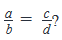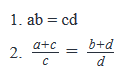Test: Ratio And Proportion- 1 - Question 5

A packet X contains pens, pencils and erasers in the ratio 1:2:3. Packet Y contains pens and pencils in the ratio 4:5.  If the total number of pens and pencils in both the packets combined is 13 and 20 respectively. What is the number of erasers in Packet X?

Detailed Solution for Test: Ratio And Proportion- 1 - Question 5

Let the number of pens, pencils and erasers in packet X be x, 2x, and 3x, respectively.

Likewise, we can let the number of pens and pencils in packet Y be 4y and 5y. Therefore, we have:
x + 4y = 13
and
2x + 5y = 20
Multiplying the first equation by 2 and subtracting that from the second equation, we have:

⇒ -3y = -6

⇒ y = 2

So x + 2(4) = 13 and hence x = 5.

Since the number of erasers is 3x, the number of erasers is 3(5) = 15.

Test: Ratio And Proportion- 1 - Question 6

Peter has an export business and he makes a profit of \$12 for every \$80 worth of goods that he exports to Europe.  If the currency in Europe is Euro and he exported goods of a total worth of 51000 Euros in the year 2011 and 1 dollar is approximately equal to 0.75 Euros, then what is his profit in 2011 in dollars(\$)?

Detailed Solution for Test: Ratio And Proportion- 1 - Question 6

Step 1: Question statement and Inferences

In order to find his profit in 2011 in dollars, we are going to follow the following procedure:

(a) Finding how many dollars is 1 Euro.

(b) Convert the worth of his goods, which is 51000 Euros into dollars

(c) Then finding profit from his worth of goods in dollars.

Step 2: Finding required values

Given:

\$1 = 0.75 Euros

Converting 0.75 into the fractional form,

\$1 = 3/4 Euros

1 Euro = \$4/3

In order to convert 51000 Euros into dollars, multiplying both sides of the equation by 51000, we get

51000 Euros = \$4/3 × 51000

51000 Euros = \$ 68000

Step 3: Calculating the final answer

In order to find out Peter’s profit by selling \$68000 worth of goods, let’s find out his profit by selling \$1 worth of goods.

\$80 worth of goods -> \$12 profit

\$1 worth of goods -> \$ 12/80 profit

\$1 worth of goods -> \$ 3/20 profit

\$68000 worth of goods -> \$ 3/20 × 68000 profit

\$68000 worth of goods -> \$10200 profit

Test: Ratio And Proportion- 1 - Question 7

For the team of salespeople in organization X, the amount of incentive provided (I) was found to be directly proportional to the sales in dollars (S) and the sales were found to be directly proportional to the Job satisfaction index (J). If the sales in the month of March of a certain year were \$30000, find the value of the job satisfaction index in March?

(1) J = 0.5 whenever S = \$10000

(2) I = 100 whenever S = \$5000

Detailed Solution for Test: Ratio And Proportion- 1 - Question 7

Step 1 & 2: Understand the question and draw inferences

Since I is directly proportional to S, we can write:

I = nS, where n is a constant . . . Equation 1

Since S is directly proportional to J, we can write:

S = mJ, where m is a constant . . . Equation 2

When S = 30,000:

mJ = 30,000

Thus, in order to find the value of J at S = 30,000, we need to know the value of m.

Step 3: Analyse statement 1

As per Statement 1,

J = 0.5 whenever S = \$10000

From Equation 2:

10000 = m(0.5)

From this equation, we will be able to find a unique value of m

Therefore, Statement 1 is sufficient

Step 4: Analyse statement 2

As per Statement 2,

I = 100 whenever S = \$5000

From Equation 1,

100 = n(5000)

From this equation, we will find the value of n. We will however, have no clue about the value of m.

Therefore, Statement 2 is not sufficient.

Step 5: Analyze statements 1 & 2 together (if needed)

Since we have obtained an answer in Step 3, there is no need to combine the statements.

Test: Ratio And Proportion- 1 - Question 8

The current manager to Direct Report ratio in a company is 1:10. If 50 more Direct Reports are added, the ratio decreases by 50%. How many managers does the company have?

Detailed Solution for Test: Ratio And Proportion- 1 - Question 8

Step 1: Question statement and Inferences

Let the number of managers in the company be M

And the number of Direct Reports be D

It is given that M/D = 1/10

⇒  D = 10M ... Equation 1

When 50 more Direct Reports are added,

Number of Direct Reports = D’ = D + 50

So, the new manager: Direct Report ratio is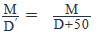Step 2: Finding required values

It is given that the new ratio is 50% less than the original ratio.

⇒ New Ratio = Original Ratio – 50% of Original Ratio

⇒ New Ratio = ½ (Original Ratio)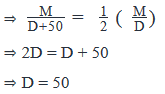Step 3: Calculating the final answer

From Equation 1,

50 = 10M

⇒  M = 5

Therefore, the company has 5 managers.

Looking at the answer choices, we see that Option B is correct

Test: Ratio And Proportion- 1 - Question 9

Three persons named A, B and C work in succession to build a machine. At first, Person A works alone and builds 1/3rd of the machine and then leaves. After person A leaves, person B works alone and builds 1/4th of the remaining machine and then leaves. After person B leaves, person C works alone and builds 1/5th of the remaining machine and then leaves. What fraction of the machine remains to be built?

Detailed Solution for Test: Ratio And Proportion- 1 - Question 9

Step 1: Question statement and Inferences

Assume the amount of work, which is to build the machine, be M. The work has been done in stages.

Step 2: Finding required values

Work done by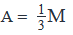Note that ‘B’ finishes a fraction of the remaining work. Therefore, we must the find work remaining at this stage.

Remaining work =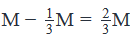Work done by B =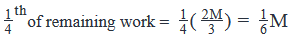Note that ‘C’ finishes a fraction of the remaining work. Therefore, we must find the work remaining at this stage.

Remaining work =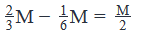Work done by C = 1th/5   of remaining work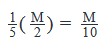Remaining work =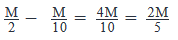Step 3: Calculating the final answer

The fraction of machine that remains to be built =RemainingworkTotalwork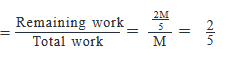Test: Ratio And Proportion- 1 - Question 10

A bag contains only two types of coins: 10 cent coins or 1 dollar coins and number of 10 cent coins does not exceed the number of 1 dollar coins. How many 10 cent coins are there in the bag?

(1) The total amount of money in the bag is \$16.50.

(2) If five 10-cent coins are removed and replaced with five 1-dollar coins then two-third of all the coins in the bag are 1 dollar coins.

Detailed Solution for Test: Ratio And Proportion- 1 - Question 10

Steps 1 & 2: Understand Question and Draw Inferences

If the number of 10-cent coins are ‘x’ and the number of 1-dollar coins is ‘y’, then we are told that x is less than or equal to y. The question is: what is the value of x?

Also, note that since ‘x’ and ‘y’ are number of coins, they must be non-negative integers.

Step 3: Analyze Statement 1

The total money in the bag due to 10-cent coins = 10x cents

The total money in the bag due to 1-dollar coins = y dollars or 100y cents

Total money in the bag = Total money due to 10-cent coins + Total money due to 1-dollar coins

16.50 * 100 = 10x + 100y

1650 = 10x + 100y

165 = x + 10y

This equation can have several integral solutions such as

if x = 5, y = 16

if x = 15, y = 15

if x = 25, y = 14, and so on.

Since x is less than equal to y, only the first two solutions are possible. ‘x’, whose value has to be found, can be 5 or 15.

INSUFFICIENT.

Step 4: Analyze Statement 2

If five 10-cent coins are replaced with five 1-dollar coins then the number of 10-cent coins become x – 5 and the number of 1-dollar coins become y + 5.

Then, y+5 = 2/3 * (x+y)

3y + 15 = 2x + 2y

y = 2x -15 ------(1)

We have to remember that x and y can only take non-negative integral values and x≤y

Substituting y from equation (1) in the given inequality, we have

x≤2x−15x≥15

x can have any value greater than or equal to 15.

For example,

if x = 15, then y = 2(15) – 15 = 15

if x = 16, then y = 2(16) – 15 = 17

and so on.

INSUFFCIENT.

Step 5: Analyze both statements together (if needed)

According to the information provided in Statement 1, x = 5 or x = 15.

According to the information provided in Statement 2, x = 15 or x = 16 or x = 17 and so on.

The common solution from the two statements is that x = 15

SUFFICIENT.

Test: Ratio And Proportion- 1 - Question 11

Kate plays a game called ‘Fruitball’ in which a fruit is thrown for the player to catch. The fruit that is thrown can be an apple or an orange. A total of 24 fruits are thrown to each player and every time a player catches a fruit, a score of ‘1’ is awarded and every time a fruit is missed a score of ‘0’ is awarded. Kate scores 2/3 of her total points from catching apples and 6 more oranges were thrown at her than apples. If the number of apples that she misses is 1/4 the number of oranges she misses, how much does Kate score in this game of ‘Fruitball’?

Detailed Solution for Test: Ratio And Proportion- 1 - Question 11

Step 1: Question statement and Inferences

Let’s say that P number of apples are thrown at her.

Then, the number of oranges thrown at her = 24 – P

Step 2: Finding required values

Since 6 more oranges are thrown at her than apples,

24 – P = P + 6

P = 9

Therefore, 9 apples and 15 oranges are thrown at her.

Let’s say that Kate catches x apples out of 9 apples thrown at her and y oranges out of 15 oranges thrown at her.

Then, her total score = x + y

If 2/3 of her total score is from catching apples, then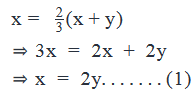The number of apples missed = 9 – x

The number of oranges missed = 15 – y

Then, since the number of apples she missed is 1/4 of the number of oranges she missed,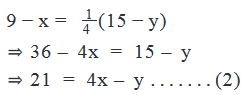Solving equation (1) and (2):

Substituting x from equation (1) in equation (2):

21 = 4(2y) – y

21 = 7y

y = 3

Step 3: Calculating the final answer

Substituting the value of y in equation (1),

x = 2 * 3 = 6

Kate’s total score at this game = x + y = 6 + 3 = 9.

Correct Choice: D

Test: Ratio And Proportion- 1 - Question 12

Peter gifted a packet of chocolates to each of the guests who came to his birthday party. Each packet was identical and contained x chocolates of flavor A, y chocolates of flavor B and z chocolates of flavor C, where x, y and z are positive integers. If the total number of chocolates in a packet was not greater than 50, then how many guests attended his birthday party?

(1) Peter used a total of 21 chocolates of flavor A, 28 chocolates of flavor B and 42 chocolates of flavor C to prepare all the packets.

(2) The numbers of chocolates of flavors A, B and C in each packet were in the ratio  3: 4: 6 respectively.

Detailed Solution for Test: Ratio And Proportion- 1 - Question 12

Steps 1 & 2: Understand Question and Draw Inferences

Notice that:

(i) Each packet contains x, y and z number of chocolates of flavor A, B and C respectively.

(ii) The packet cannot hold more than 50 chocolates. In other words, x + y + z ≤ 50

If N friends come to his party, then the total number of flavor A, flavor B and flavor C chocolates are Nx, Ny and Nz respectively.

Notice that the total number of chocolates of flavor A, flavor B and flavor C have at least one common factor: N, the number of friends who attended his party.

Step 3: Analyze statement 1

The numbers 21, 28 and 42 represent the total number of flavor A, flavor B and flavor C chocolates.

=> Nx = 21

Ny = 28

Nz = 42

The common factors of Nx, Ny and Nz are 1 and 7.So, the number of friends could be 1 or 7.

Case I: N = 1

=> x = 21, y = 28, z = 42.

=> x + y + z = 91

Since x + y + z > 50, this case is not possible.

Case II: N = 7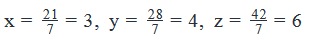x + y + z = 3 + 4 + 6 = 13, which satisfies x + y + z ≤ 50.

This is the only possible case. Therefore, N = 7.

SUFFICIENT.

Step 4: Analyze statement 2

Since the ratio of the number of chocolates of flavor A, flavor B and flavor C is 3:4:6, we can assume the number of chocolates of flavor A, flavor B and flavor C to be 3k, 4k and 6k respectively. (k can be any positive integer)

If the number of friends who attended Peter’s birthday party is N, then the number of chocolates of flavor A, flavor B and flavor C are 3k×N, 4k×N and 6k×N respectively.

There is no information relating 3kN, 4kN and 6kN, and therefore N cannot be found.

INSUFFICIENT.

Step 5: Analyze Both Statements Together (if needed)

Since we have obtained an answer in Step 3, there is no need to combine the statements.

Test: Ratio And Proportion- 1 - Question 13

If two inches are approximately equal to 5 centimeters, how much is the area of a painting in square inches if its area is 2900 square centimeters?

Detailed Solution for Test: Ratio And Proportion- 1 - Question 13

Step 1: Question statement and Inferences

We have been provided the relation between inches and centimeters. We have to convert an area of 2900 square centimeters into square inches.

We shall follow the following procedure:

(a) First find out, by the Unitary Method, how many inches are there in 1 centimeter.

(b) Then, find out how many square inches are there in 1 square centimeter.

(c) Lastly, find out how many square inches are there in 2900 square centimeters, which is the required answer.

Step 2: Finding required values

Given: 2 inch = 5 centimeters

Writing this equation in reverse, we get:

5 centimeters = 2 inches

=> 1 centimeter = 2/5 inch ----------------(1)

1 square centimeter = 1 centimeter X 1 centimeter

In order to find out how many square inches is 1 square centimeter, substituting the value of 1 centimeter from equation (1) in the above equation, we get

1 square centimeter = 2/5 inch * 2/5 inch

Since inch*inch is square inches,

1 square centimeter = 4/25 square inches

Step 3: Calculating the final answer

In order to find out how many square inches is 2900 square centimeters, multiplying both sides of the above equation by 2900,

2900 square centimeters = 4/25 * 2900 square inch

2900 square centimeters = 464 square inches

Test: Ratio And Proportion- 1 - Question 14

If a : b = 7 : 5, b : c = 9 : 11, find a : b : c?

Test: Ratio And Proportion- 1 - Question 15

If a: b = 3:4, b:c = 7:9, c:d = 5:7, find a:d?

Detailed Solution for Test: Ratio And Proportion- 1 - Question 15

a/d = (3/4)*(7/9)*(5/7) => 5/12

## Quantitative for GMAT

88 videos|99 docs|165 tests
 Use Code STAYHOME200 and get INR 200 additional OFF Use Coupon Code
Information about Test: Ratio And Proportion- 1 Page
In this test you can find the Exam questions for Test: Ratio And Proportion- 1 solved & explained in the simplest way possible. Besides giving Questions and answers for Test: Ratio And Proportion- 1, EduRev gives you an ample number of Online tests for practice

## Quantitative for GMAT

88 videos|99 docs|165 tests